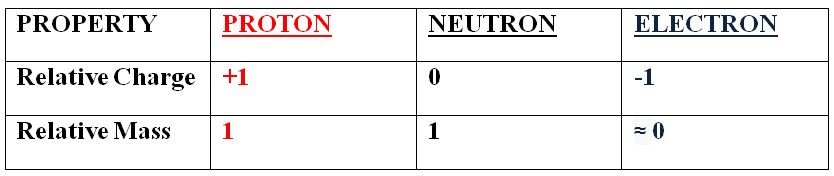# Atomic structure

### Atomic structure

#### Lessons

In this lesson, we will learn:
• The definition of an atom.
• The particles that make up an atom and where in the atom they are found.
• The charge and mass of an atom's constituent particles.

Notes:

• The atom is the smallest indivisible unit of matter, comprised of protons, neutrons and electrons.

• It's known as the smallest indivisible unit of matter because an atom is the smallest unit of a material that still shows all the properties of the bulk material. It is also the smallest unit of matter that is stable in its own right.

• Atoms are comprised of three main particlesprotons and neutrons, which make up the central core of the atom known as the nucleus, and electrons, which are found in shells (or orbitals) occupying space outside the nucleus.

• A brief overview of the particle properties is below:• The nucleus contains almost all of the mass of an atom as it contains protons and neutrons, which both have a relative mass of 1 AMU. The nucleus also has an overall positive charge, as neutrons have no charge and protons have a relative charge of +1.

• The mass of an electron is (relative to protons and neutrons) so small that it is often ignored; it is around 1/1840 AMU. An electron has a relative charge of -1 so an electron has an equal but opposite charge to a proton.

• In an atom of an element, the number of protons is always equal to the number of electrons. This means the overall charge of an atom is zero and is therefore neutral.

• Because the atomic mass of an electron is approximately 0, we can say that the atomic mass, or mass number, of an element tells us the number of protons and neutrons in an atom because only these two particles contribute to the mass of the atom.

• Using the last two points of information, we can look at the periodic table and predict the number of protons, electrons and neutrons in an atom of any element!

• Introduction
Introduction to atomic structure
a)
Inside an atom.

b)
The particles that make an atom

c)
Mass and charge of particles

d)
Predicting elements.

• 1.
Use information on elements in the Periodic Table to identify the number sub-atomic particles in elements.
Find the number of protons, neutrons and electrons in an atom of the following elements:
a)
i) Nitrogen
ii) Silicon

b)
i) Calcium
ii) Aluminum

• 2.
Apply knowledge of atomic number and mass number in the Periodic Table to identify elements.
Use the information in each statement to find the identity (element) of each atom:
a)
i) A neutral atom with 9 protons.
ii) An atom known to have 10 neutrons and an atomic mass of 20

b)
i) A neutral atom with 48 electrons
ii) An atom which, when it contains 10 electrons will have an overall charge of -2